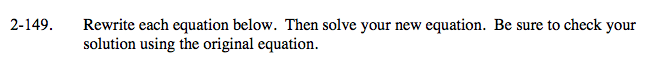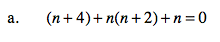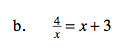### Home > AC > Chapter 13 > Lesson 13.RE3-S > Problem2-149

2-149.Distribute the n.

Combine like terms, and factor the equation.

Simplify by Zero Product Property, then subtract 2 from both sides.

n + 4 + (n2 + 2n) + n = 0

n2 + 4n + 4 = 0

(n + 2) (n + 2) = 0

n + 2 = 0

n = −2Multiply both sides by x. Then set the equation equalto 0 and use the ZPP.

x = −4 or x = 1